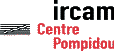Serveur © IRCAM - CENTRE POMPIDOU 1996-2005.Tous droits réservés pour tous pays. All rights reserved.

# Density of spectral components : preliminary experiments

Andrew Gerzso

Rapport Ircam 31/80, 1978

## Abstract

How many sinusoids must one accumulate in a frequency band to produce a signal perceptually equivalent to a noise signal of the same bandwidth ?

Using forced choice technique, subjects matched a band of noise approximately a critical bandwidth wide with another band of equal width which was progressively filled with random phased, equal amplitude sinusoids whose frequencies randomly but uniformly filled out the band.

The bandwidths chosen were : 100-175 Hz, 400-510 Hz,1270-1480 Hz, 3700-4400 Hz. The number of sinusoids varied between 3 and 25. For the first three bandwidths findings show the point of subjective equivalence (PSE) to occur in the neighborhood of 15 sinusoids.

Room acoustics seem to play an important role in determining the PSE. Fewer sinusoids are needed in a reverberant environment than in a dry one.

## Introduction

These experiments were designed to provide preliminary answers to the following questions. To what extent are sounds of different spectral densities (i.e. varying numbers of sinusoidal components per given bandwidth) perceptually distinguishable ? Is there a threshold beyond which differences of density are no longer perceivable ?

The answers to these questions are of interest not only to those who are concerned with developing a theory of hearing, but also to those who are affected by the practical implications, such as modern instrument builders and composers. A synthesizer builder may want to know how many oscillators he needs to effectively cover the auditory range. A composer interested in complex vertical structures may want practical information regarding the perceptibility of these structures. Recently, spectral density was a topic of discussion in a series of lectures by Pierre Boulez (1).

The idea of spectral density is not new. Experiments on the frequency selectivity of the ear carried out by Schafer et al. (2) made use of synthetic noise in their masking experiments. The synthetic noise was constructed by adding large numbers of sinusoidal components. Tests to determine the number of sinusoids needed to simulate a 32 Hz band of noise showed that a frequency spacing of 1 Hz was necessary. Their work implied, of course, that more sinusoids were needed to synthesize noise for wider bands than for narrower bands. Here the experimental paradigm differed from that of Schafer et al. only in the bandwidths chosen for the noise. Since this experiment was in fact concerned with the resolving power of the ear and therefore the auditory filter (critical band), the bandwidths were measured in barks. And so the bandwidth used here was always one bark (one critical bandwidth) based on the data provided by Scharf (3).

Some of the experimental findings were, of course, unexpected and not immediately obvious. For this reason and because of practical considerations of presentation, the results are not presented in the chronological order of the actual tests. Instead, an attempt is made to present the basic findings in a logical fashion on an issue by issue basis.

As shall be seen below, reverberation and roughness play important roles in the determining the PSE.

## The rooms

The tests took place in two rather different rooms. The first was a conference room which shall be called the large room (LR). It measured approximately 8 m. by 15 m. and contained many reflecting surfaces (metal cabinets and bookcases, glass wall at the rear, concrete walls on the sides, iron doors), but also an absorbing surface (carpeted floor). At the time of the tests there were about 35 people in the room not all of whom actually took the tests. The tests themselves were on tape (Ampex 456 1/4 inch) played over two loudspeakers (Phillips DRH545) placed in front and to the left and right of the subjects using a tape recorder (Revox A77 equipped with DBX noise reduction). The average distance between the subjects and the loudspeakers was 6 m.

The second room was a small studio which shall be called the small room (SR). It measured approximately 5 m. by 4 m. and contained many absorbing surfaces (cloth covered walls, carpet). The average distance between the loudspeakers and subjects was 1.5 m.

## The stimuli

All stimuli described below were generated numerically using a program running on a Digital Equipment Corporation DEC-10 computer. All signals were generated for Digital-to-Audio converters with 16 bit amplitude resolution at a 25641 Hz sampling rate. A smoothing filter with a cutoff followed the output of the converter. Two types of noise were made.
1. Band limited noise (BLN).
2. Synthetic noise (SN) by the addition of sinusoids.
All stimuli were matched for equal subjective loudness.

The band-limited noise was produced by generating normally distributed random numbers which were computed by the exact method described by Knuth, using Algorithm P, the Polar method for Normal deviates (4). The noise was then filtered by a 12th order bandpass filter which was simulated digitally, thus giving a 36 dB per octave rolloff on each skirt. The filter was calculated from the Butterworth approximation which was chosen for its relatively low level of ringing. The Butterworth filter was then transformed to the digital domain by use of the bilinear transform, taking into account the frequency transformation necessary to make the 3dB points lie exactly at the desired frequencies. Informal listening tests with higher order bandpass filters indicated little or no change in the sound quality with increase in order beyond 12. In all cases the signals were 1.5 S long with rise and decay times of 20 msec. The program to make the BLN was written in the programming language SAIL by James A. Moorer.

The synthetic noise (SN) was produced using the MUSIC-10 program from Stanford University. The space between the upper and lower limits of the critical band was divided logarithmically by the number of sinusoids desired in the space. Each frequency thus obtained was then multiplied by .003 to obtain a number which when added and subtracted from the original frequency would provide the upper and lower limits within which a random number was chosen. In this way the space within the critical band was filled out in a uniform but random fashion. All sinusoids were random phased. The amplitude of each sinusoid was calculated by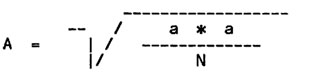where A is the amplitude of each sinusoid, a the maximum amplitude available in the Music 10 program, and N the total number of sinusoids. As with the BLN, each SN was 1.5 S long with rise and decay times of 20 msec.

## Test procedure

Four different critical bands were chosen for study : 100-175 Hz, 400-510 Hz, 1270-1480 Hz, and 3700-4400 Hz.

The test for each critical band was carried out in the following manner. The test began with a warning signal consisting of four short beeps at intervals of .5 S. The subject was then presented 19 times with the task described next. Following a warning beep the subject heard three noises (two BLN and one SN) separated by pauses of .5 seconds. The subject then had to say which of the three noises was the SN. Five seconds were provided to write down the answer. At the end of the five seconds the warning beep for the next task was heard and so forth. During each test, therefore, the subjects heard a total of 57 noises (19 SN and 38 BLN). Each BLN was different in order to avoid giving cues through repetition. Each of the 19 SN contained a different number of sinusoids. The SN contained from 3 to 25 sinusoids. (These figures were arrived at through informal listening tests.) The position of the SN in each task was chosen at random. Furthermore, the number of sinusoids in each SN was randomly varied task by task.

In two of the tests the BLN was replaced in each task by SN containing 100 sinusoids. This type of noise shall be designated SN100 to distinguish it from the SN containing 3, 5, 7, 9 .... 25 sinusoids. As with the BLN, 38 different SN100 were made for each test in which the SN100 was used.

As mentioned before, each test was done with a tape which was played over two loudspeakers in front of the subjects. The level for each of the tests was approximately 80 dB. The subjects were both male and female with an average age of 35 years. About half were musicians.

When several tests were done consecutively in one testing session, a rest period of about 2 minutes was given between each test. The largest number of consecutive tests was 5, which made for a total testing time of about 25 minutes. There were four testing sessions with different subjects each time.

The chronological order of the tests may be seen in Table I which gives for each test the result code number, bandwidth, room, type noise used (BLN or SN100), number of subjects, number of times the test was done, and the number of the testing session.

## Results

The results may be observed in Figs.1-9. The percentage of correct answers is on the ordinate axis, and the number of sinusoids on the abscissa. The 33% chance performance level line is also drawn.

These raw data were fit with a simple exponential curve of the form

Ae-Bk + .333
(where k is the number of sinusoids) by a nonlinear least squares optimisation program which used the Marquart iteration. The parameters A and B were thus adjusted to minimize the error between the exponential curve and the experimental data. The curve fitting program was written in SAIL by James A. Moorer. Figs. 1, 2, and 3 reveal three interesting facts. (In the discussion below we shall adopt an arbitrary figure of 50% correct for the PSE.)
1. In all cases subjects gave more correct answers in the acoustically dry SR than in the more reverberant LR.

2. In the LR the PSE increases from approximately 6 to 12 sinusoids for the three critical bands studied. An average of 10. In the SR the PSE increases from 10 to 20 sinusoids. An average of 16. F-tests on the LR and SR data, however, do not show a significant amount of variance, so for all practical purposes we may consider that the PSE occurs in approximately the same place in the SR and the same place in the LR in all three critical bands. In comparing the SR data with the LR data the Mann-Whitney U Test at the .025 probability level revealed a statistically siginificant difference for the first and third critical bands studied. We may conclude from this that the room acoustic plays a role in determining the PSE.

3. The number of sinusoids needed to simulate the BLN seems to be approximately the same for the three critical bands studied. This suggests that perhaps the 1 Hz spacing needed by Schafer et al. to simulate band limited noise was, in fact, not necessary. According to their results the first band studied would need 75 sinusoids, the second 110 sinusoids, and the third 210 sinusoids. Here, in the case of the LR, approximately 13 to 20 sinusoids are needed in each of the critical bands to simulate the BLN.
The test of the 100-175 Hz critical band was repeated after the subjects had taken 4 other tests. The results are shown in Fig. 4. It would appear that a modest improvement in performance took place. However, the Mann-Whitney U Test does not support this conclusion.

Fig. 5 compares the results for the 3700-4400 Hz critical band with the three others discussed so far. It is immediately obvious that there is a very noticeable difference in performance. At first it was thought that the subjects might be getting some kind of spectral cue from the BLN which made it easy to choose the correct position of the SN. Following a suggestion by Max Mathews, the test was repeated with the SN100 replacing the BLN. The results of that test together with the test using the BLN are shown in Fig. 6. The results are nearly identical. It should be noticed that the test with the SN100 was done in the SR. In contrast to the results in Figures 1, 2, and 3, the difference in room acoustic seems to make no difference in the performance of the subjects.

Fig. 7 compares the results of the 400-510 Hz critical band using on the one hand the BLN and on the other the SN100. The performance in each case is very similar. This would seem to suggest that in fact there is no substantial difference between using the SN100 and the BLN.

Fig. 8 shows the effect of narrowing the 3700-4400 Hz critical band by 100 Hz to 3700-4300 Hz. There is a clear decrease in performance on the part of the subjects. The curve for this poorer performance is actually very close to the SR results for the first three critical bands studied.

Fig. 9, in contrast to Fig. 8, shows the effect of widening the 1270-1480 Hz critical band by 90 Hz to 1270-1570 Hz. Widening the band seems to improve performance.

## Discussion

Discussions with the subjects at the end of each testing session revealed that roughness played a very important role in deciding which of the three noises was the SN. Once having gotten an idea of the shape and regularity of the amplitude fluctuations of the three noises, the subject would invariably choose the noise that had the greatest fluctuations. Also, any stimulus that had a regular beating pattern would be chosen. It is likely that when the roughness resembled that of the BLN, it became difficult for the subject to make the correct choice

Recent experiments by Terhardt (5), (6), (7) have revealed two important facts regarding roughness.

1. Roughness is determined by amplitude fluctuations.
2. As the fluctuations increase in speed the ear has a harder and harder time following them.
Experiments, by the same author using amplitude modulated tones (AM tones), have shown that the bandwidth at which roughness dissappears is different for frequencies < 2000 Hz than for frequencies > 2000 Hz. For convenience we shall call the bandwidth at which roughness disappears the ROB (roughness disappearance bandwidth). For frequencies < 2000 Hz the ROB is equal to the critical bandwidth. For frequencies > 2000 Hz the ROB is less than the critical bandwith with a constant value of about 250 Hz.

Other experiments measuring the roughness of two beating tones by Plomp and Steeneken (8) are generally in agreement with those of Terhardt. For frequencies > 3000 Hz the ROB is less than the critical bandwidth. At 4000 Hz for example the ROB is approximately 400 Hz whereas the critical bandwidth in this region is approximately 700 Hz.

It will be recalled that the results shown in Figs. 1, 2, and 3 showed that approximately the same number of sinusoids were needed in the SN to simulate the BLN in each of the bandwidths studied. This suggests a strong correlation between the number of sinusoids needed and the critical bandwidth or the ROB which in the regions studied, is equal to the critical bandwidth.

Fig. 6 showed a very high performance for the 3700-4400 Hz band in both the LR and the SR. The fact that this bandwidth of 700 Hz exceeds the ROB in both studies mentioned above may explain why the results were so different for the highest bandwidth studied. In order to have had performance levels comparable to the ones in Figs. 1, 2, and 3, it is likely that for the highest band studied the bandwidth should have been smaller and perhaps close to either of the ROB figures given above. The results in Fig. 8 for the narrowed band would tend to support this likelihood.

If in fact the number of sinusoids needed to simulate BLN is correlated with the RDB, as the present study would seem to suggest, then the number of sinusoids needed per critical band would be the same in the region below 2000 Hz, and for each successive critical band above 2000 Hz the number would increase.

Figs. 1, 2, and 3 would seem to suggest that reverberation plays a role in determining the spectral density saturation threshold. Since the SR was considerably less reverberant than the LR it is likely that the subjects were able to hear more clearly the amplitude fluctuations and therefore be in a better position to give a correct answer.

On the other hand the objection might be made that in Fig. 5 the SR did not improve the performance for the 3700-4400 Hz band. The issue is further complicated by the fact that the test in the SR was done with a different kind of noise (the SN100). It will be recalled that Fig. 7 shows that there is a great similarity in performance when either the BLN or the SN100 is used. Furthermore, as shown in Fig. 9, widening the bandwidth improves performance. In the light of these two points it would not be unreasonable to suggest that the similarity of performance was due to the fact that the bandwidth was wider than the ROB (by a factor of 2) and that therefore the subjects were presented with relatively easy tasks where reverberation did not play a very large role.

## Conclusions

As a result of the preliminary experiments on spectral density undertaken above, the following tentative conclusions might be drawn.
1. A correlation appears to exist between the number of sinusoids needed to simulate BLN, and the ROB or critical bandwidth for frequencies less than 3000 Hz. Also, reverberation appears to play a role in determining the PSE. The more reverberation the lower the threshold. In a moderately reverberant room such as the LR, roughly 16 sinusoids of equal amplitude are needed per critical band or ROB to simulate BLN.

2. Assuming that a correlation exists between the number of sinusoids needed to simulate BLN, and the ROB for frequencies > 3000 Hz, roughly the same number of sinusoids as above would be needed per ROB. However, for each successive critical bandwidth the number would be higher. For example, for the 3700-4400 Hz critical band, assuming an ROB of 400 Hz and 20 sinusoids per ROB, the number of sinusoids needed would be roughly 35. For the 8000-9600 Hz critical band, assuming an ROB of 750 Hz, the number of sinusoids needed would be roughly 43.

## Future works

Further work using the same experimental paradigm should be undertaken to clarify some of the results shown in this paper. It would be advantageous to repeat the experiments in rooms where the response characteristics are known in a more objective manner in order to get a better idea of the role played by reverberation in determining the PSE. Monaural tests with earphones should also be done. More tests should be done in the > 3000 Hz frequency range in order to clarify whether the correlation exists between the ROB or critical band, and the number of sinusoids needed to simulate BLN.

## Acknowledgements

The author is very indebted to Pierre Boulez for permitting the first of the experiments to take place during one of his Seminaires for the College de France. To Max Mathews for support for the work undertaken and conversations rich in sound advice and insight. To Andy Moorer for his noise and curve fitting programs and friendly help. To Johann Sundberg for important advice and criticism. To David Wessel for illuminating discussions on critical bands and methods in experimental psychology. To all the kind people at IRCAM who helped directly or indirectly in the realization of this project.

## Table I

Code number Bandwidth Room Noise used Num. subj. Num. times test done Test session number
R1 100-175 Hz LR BLN 14 1 1
R2 400-510 Hz LR BLN 14 1 1
R3 1270-1480 Hz LR BLN 14 1 1
R4 3700-4400 Hz LR BLN 14 1 1
R5 100-175 Hz LR BLN 14 1 1
R6 3700-4400 Hz SR SN100 7 1 2
R7 400-510 Hz SR SN100 13 1 2
R8 3700-4300 Hz SR BLN 7 1 3
R9 1270-1570 Hz SR BLN 7 1 3
R10 100-175 Hz SR BLN 5 1 4
R11 400-510 Hz SR BLN 5 1 4
R12 1270-1480 Hz SR BLN 5 1 4

#### FIGURE 1

Percentage of correct responses as a function of the number of sinusoids per bandwidth studied. Comparison of LR and SR.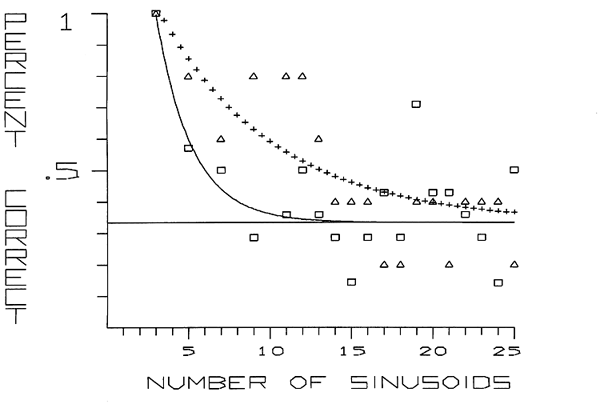Code number Symbol Bandwidth Noise used Num. subj. Room
R1 ---- 100-175 Hz BLN 14 LR
R10 ++++ 100-175 Hz BLN 5 SR

#### FIGURE 2

Percentage of correct responses as a function of the number of sinusoids per bandwidth studied. Comparison of LR and SR.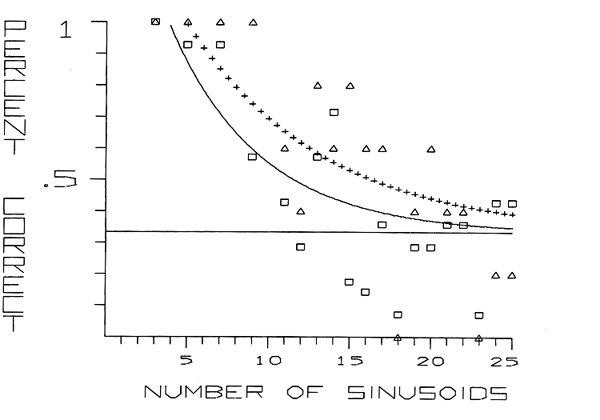Code number Symbol Bandwidth Noise used Num. subj. Room
R2 ---- 400-510 Hz BLN 14 LR
R11 ++++ 400-510 Hz BLN 5 SR

#### FIGURE 3

Percentage of correct responses as a function of the number of sinusoids per bandwidth studied. Comparison of LR and SR.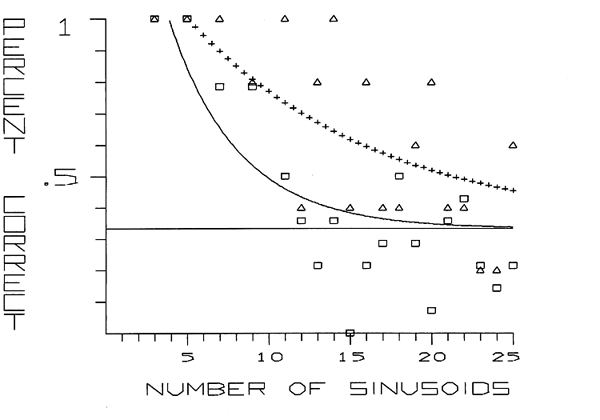Code number Symbol Bandwidth Noise used Num. subj. Room
R3 ---- 1270-1480 Hz BLN 14 LR
R12 ++++ 1270-1480 Hz BLN 5 SR

#### FIGURE 4

Percentage of correct responses as a function of the number of sinusoids per bandwidth studied. The learning effect.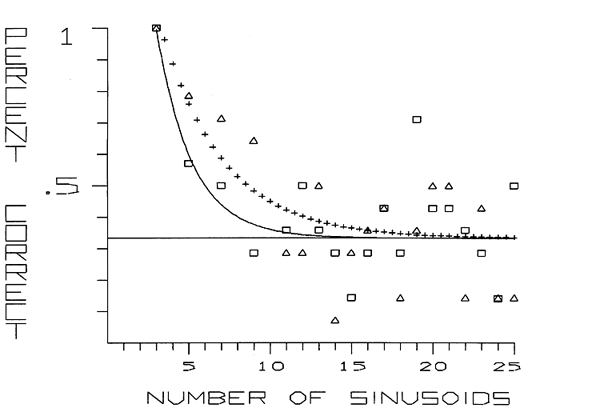Code number Symbol Bandwidth Noise used Num. subj. Room
R1 ---- 100-175 Hz BLN 14 LR
R5 ++++ 100-175 Hz BLN 14 LR

#### FIGURE 5

Percentage of correct responses as a function of the number of sinusoids per bandwidth studied. Comparison of the results for the LR of Figures 1-3 and the highest critical bandwidth studied.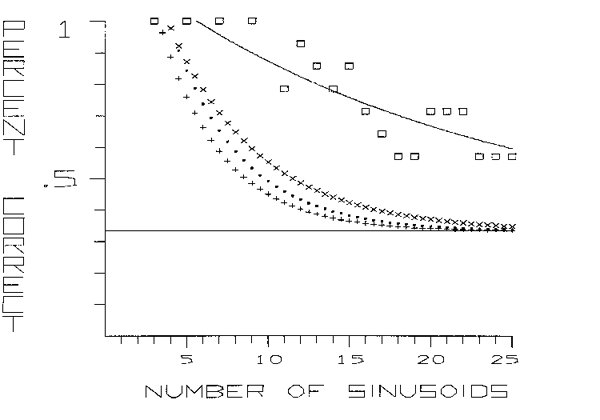Code number Symbol Bandwidth Noise used Num. subj. Room
R4 ---- 3700-4400 Hz BLN 14 LR
R5 ++++ 100-175 Hz Hz BLN 14 LR
R2 xxxx 400-510 Hz BLN 14 LR
R3 .... 1270-1480 Hz BLN 14 LR

#### FIGURE 6

Percentage of correct responses as a function of the number of sinusoids per bandwidth studied. Comparison of BLN and SN100.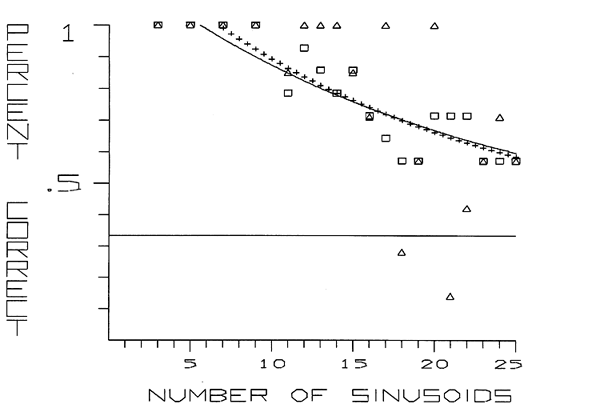Code number Symbol Bandwidth Noise used Num. subj. Room
R4 ---- 3700-4400 Hz BLN 13 LR
R6 ++++ 3700-4400 Hz SN100 7 SR

#### FIGURE 7

Percentage of correct responses as a function of the number of sinusoids per bandwidth studied. Comparison of BLN and SN100.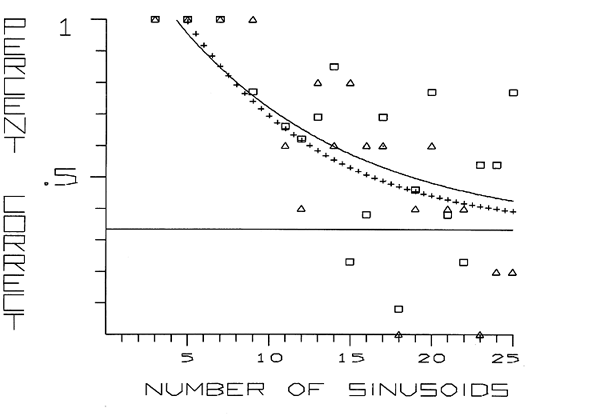Code number Symbol Bandwidth Noise used Num. subj. Room
R7 ---- 400-510 Hz SN100 13 SR
R11 ++++ 400-510 Hz BLN 5 SR

#### FIGURE 8

Percentage of correct responses as a function of the number of sinusoids per bandwidth studied. Effect of narrowing the bandwidth.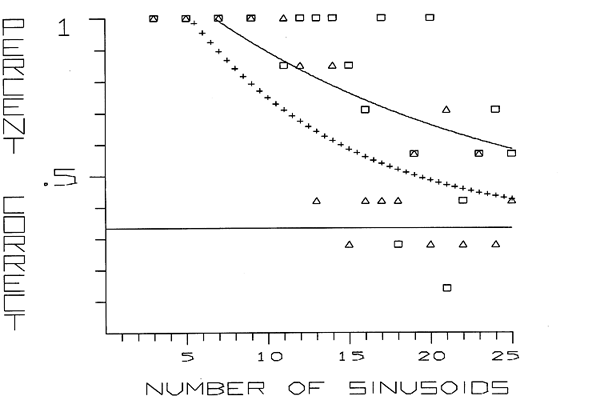Code number Symbol Bandwidth Noise used Num. subj. Room
R6 ---- 3700-4400 Hz SN100 7 SR
R8 ++++ 3700-4300 Hz BLN 7 SR

#### FIGURE 9

Percentage of correct responses as a function of the number of sinusoids per bandwidth studied. Effect of widening the bandwidth.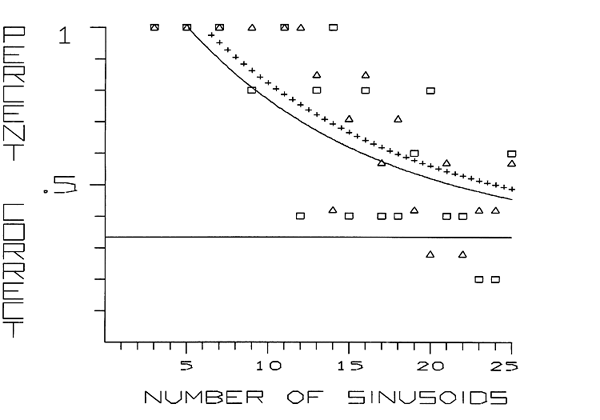Code number Symbol Bandwidth Noise used Num. subj. Room
R12 ---- 1270-1480 Hz BLN 5 SR
R9 ++++ 1270-1570 Hz BLN 7 SR

### References

(1) Boulez, P.
Séminaires du Collège de France (1978).
(2) Schafer, T.H., Gales, R.S., Shewmaker, C.A., and Thompson, P.O.
The frequency selectivity of the ear as determined by masking experiments. J. Acoust. Soc. Amer. 22,(1950),490-496.
(3) Scharf, B.
Critical Band In Tobias, J.V.(Ed.), "Foundations of Modern Auditory Theory", Vol. I. Academic Press, New York, 1970. pp.159-202.
(4) Knuth, D.E.
(5) Terhardt, E.
Uber die durch amplitudenmodulierte Sinustone hervorgerufene Horempfindung. Acustica 20 (1968), 210.
(6) Terhardt, E.
Uber akustische Rauhigkeit und Schwankungsstärke. Acustica 20 (1968), 215.
(7) Terhardt, E.
On the perception of periodic sound fluctuations. Acustica 30 (1974), 201.
(8) Plomp, R., and Steeneken, H. J. M.
Interference between two simple tones. J. Acoust. Soc. Amer. 43 (1968), 883.

____________________________
Server © IRCAM-CGP, 1996-2008 - file updated on .

____________________________
Serveur © IRCAM-CGP, 1996-2008 - document mis à jour le .